# Ricochets

### March, 1995

Calculating a ricochet turns out to be a lot trickier problem than it first appears. For those of us who are a decade or more away from our last practice with algebra and trigonometry it requires some patience and perseverance. We found some descriptions of transferring momentum between balls once the angle between them was known.  But not how to determine where the balls are at the time of the collision.  This description will step you through one way to answer those questions.

First we're going to concentrate on the collision of two balls. Our algorithm uses fairly simple algebra and trig to find the time and location of the collision to an arbitrarily high precision without expensive iteration. Later we'll expand that to the collision of a ball against a wall. Our discussion will include mass and elasticity. It does not include anything about friction, spin, or other factors needed in a high fidelity billiard sim. For that, check out the CAROM simulator in the related files. At the end we'll discuss some of our ideas on how we might handle simultaneous collisions between multiple objects.

First, two balls.

There are six parts to the calculation, with a couple of subparts.

## 1. Gross collision detection.

The purpose of this step is to determine in as few instructions as possible whether the objects are close enough together to warrant more expensive calculations. There are many ways to accomplish this. The one we chose was the simple bounding box (See figures 1a through 1c).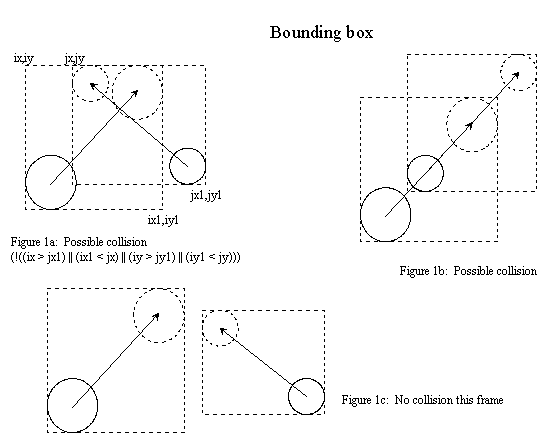The quickest way to find out if two bounding boxes overlap is to test to see if the bounding boxes DON'T overlap. It only takes four tests to show that box A is to the right of, left of, above, or below box B.

// Use this macro in an if statement. Ex.
// if (bounding_box_test(ibx, ibx1, iby, iby1, jbx, jbx1, jby, jby1))
// DoSomething();
// else
// DoSomethingElse();
#define bounding_box_test(ibx, ibx1, iby, iby1, jbx, jbx1, jby, jby1) \
(!((ibx > jbx1) || (ibx1 < jbx) || (iby > jby1) || (iby1 < jby)))

You'll need to extend the bounding boxes to include both the beginning and ending positions of each object. Otherwise, the objects could become overlapped before you detect a collision.

## 2. Calculate accurate positions at time of collision.

The central trick for this step is the Quadratic equation.

Let's take a moment to define our terms. (See figure 2a)Stuff we know:
x1,y1 x2,y2 - The initial positions of the centers of the two objects
dx1,dy1 dx2,dy2 - The distances the objects moved this frame
r1, r2 - radii of the two objects

Stuff we calculate:
t - Time of collision
cx1,cy1 cx2,cy2 - The centers at time of impact

What we're trying to find is when the centers of the two objects are the same distance apart as the sum of their radii. The distance formula is sqrt([cx1-cx2]^2 + [cy1-cy2]^2). Therefore, we're looking for the time (t) when (r1+r2)=sqrt([cx1-cx2]^2 + [cy1-cy2]^2).

How do we know where a ball will be at any given time (t)? Here are functions which describe the paths of the centers of the balls over time:

cx1 = x1 + tdx1 cx2 = x2 + tdx2
cy1 = y1 + tdy1 cy2 = y2 + tdy2

The t's relate all the forumlas to each other. Replace the cx's and cy's in the distance forumla with these equations and we end up with:

r1+r2 = sqrt(((x2 + tdx2)-(x1 + tdx1))^2 + ((y2 + tdy2)-(y1 + tdy1))^2)

Multiply everything out then rearrange and simplify and you end up with:

0 = [(dx2-dx1)^2 + (dy2-dy1)^2] t^2 +
[2(x2-x1)(dx2-dx1) + 2(y2-y1)(dy2-dy1)] t +
[(x2-x1)^2 + (y2-y1)^2 - (r1+r2)^2]

This is a polynomial in the form 0=ax^2+bx+c. We can use the Quadratic equation (-b(+|-)sqrt(b^2-4ac))/2a to solve for the variable. The a, b and c are all spelled out above. You could plug a, b and c into that monster equation, but it turns out you want to calculate it in pieces.

The Quadratic equation will give two answers, call them t1 and t2. One for each the plus and minus of the square root portion. There are some special cases to check before solving the equation, and some special cases to check after solving the equation. (See figure 2, cases 1-6)#### Special cases:

1) If a=0 the equation goes infinite. Notice that "a" consists of the square of the difference between the direction vectors. Therefore, a=0 if and only if the balls are moving the same direction and the same speed.

2) If 4ac > b^2 then you end up trying to take the square root of a negative number. In other words, there's no solution. It turns out that this happens if and only if the balls never pass within r1+r2 of each other. Even though the paths may cross, the balls are far enough apart in time that they don't approach close enough to each other to ever actually touch.

3) If both t's are negative then the balls are receding from each other.

4) If one t is negative and the other t is positive then the balls began the frame already overlapped.

5) If both t's are positive then the smaller of the two is when they first reach r1+r2 from each other.

6) If both t's are greater than 1.0 then the balls do not collide this frame.

Condition 5 is the only valid collision and you continue with the rest of the calculations.

Conditions 1, 2, 3 and 6 indicate that there is not a valid collision this frame and you therefore can abort before performing the rest of the calculations. In effect, you discard this pair of objects since a collision didn't take place.

Ideally, condition 4 should not occur. Whether or not it does occur depends on whether or not you handle multiple collisions in one frame. If you don't test for condition 4 then the rest of the math works out that the balls will remain locked together "arm-in-arm" so to speak.

## 3. Calculate the angle of collision vs the velocity vector

In order to calculate the momentum transfer later on we need to perform the remainder of these steps on both objects.

At first glance it appears that you simply take the angle between the paths of the balls. That doesn't work. Let's take the example of two balls headed in the same direction and one ball is catching up on the other. If their centers lie on the same line then it's a simple 1D collision. But if the center of one ball is offset a little to one side then the momentum transfer occurs at an angle even though the motion paths are parallel. (See figures 3a and 3b)What we need to find is the angle formed by the velocity vector and the line connecting the centers. We need this angle in order to calculate how much of the velocity vector to apply to the collision. In other words, if the balls hit head-on, as in figure 3a, then the entire velocity vector is applied to the collision. If the balls just nick each other, as in figure 3b, then less momentum is transfered between the balls.

The mathmatical tool for this problem is the Law of Cosines. a^2 = b^2 + c^2 - 2bc cos(A). As written, it's intended for finding the length of a side when an angle is known, but we can flip it around:
cos(A) = ( a^2 - b^2 - c^2 ) / (-2bc)

Thus the angle is: A = acos( ( a^2 - b^2 - c^2 ) / (-2bc) )

Note that we can't use "simple" trig since we're not guaranteed of having a right angle. (See figure 3c and 3d) This way you won't have to transform coords to some artificial origin.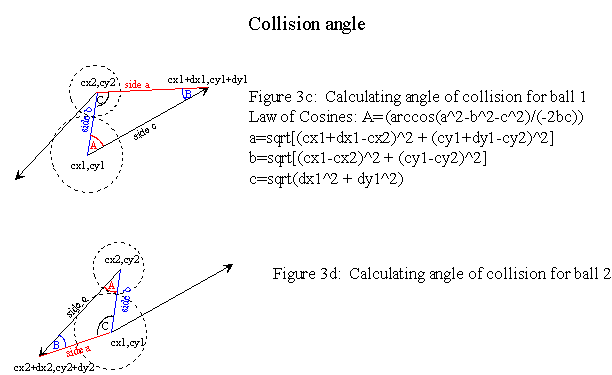(-2bc) approaches 0 if and only if the radii of both objects approach 0. That's a pretty obvious should-not-occur situation.

## 4. Calculate portion of velocity vector that applies to collision

Once we have angle A this part turns out to be trivial. The piece of the total velocity vector that points toward Ball 2 is: vi=cos(A)*sqrt(dx1^2+dy1^2)

One obvious optimization is to save and use cos(A) above, rather than find A only to immediately take its cos() again.

## 5. Calculate final velocities along collision vector

At this point we can treat this as a 1D collision along the line connecting the centers. You can find the momentum transfer equations in most first-year college physics texts.

vf1=(((m1-m2)*vi1)+(((1.0+(e1*e2))*m2)*vi2))/(m1+m2); vf2=((((1.0+(e1*e2))*m1)*vi1)+((m2-m1)*vi2))/(m1+m2);

Where:
vf1, vf2 - final velocities along collision vector
vi1, vi2 - initial velocities along collision vector
m1, m2 - mass of each ball
e1, e2 - elasticity of each ball

## 6) Apply final velocities back to the object

Now we need to subtract the off old velocity vector and add on the new one. That will leave us with an object that's moving at the new, adjusted speed after ricochet.

angle=atan2(dcy,dcx); // angle of the line connecting the centers
sina=sin(angle);
cosa=cos(angle);
odx=cosa*vi; // subtract off old velocity vector
ody=sina*vi; ndx=cosa*vf1; // add on new velocity vector
ndy=sina*vf1;
dx+=odx-ndx; // signs look reversed but are correct
dy+=ody-ndy;

So, that's a ricochet

So, that's how you ricochet two balls. Whew! It seems like a lot of work, but there's no magic involved. Just break the problem into pieces and use the appropriate tool for each piece.

We haven't discussed optimizations, yet. It turns out that collisions happen rarely enough that even using floating point and transcendental functions like sin and cos doesn't slow things down noticably. For example, an Asteroids clone with 30-40 rocks bouncing around spends almost all its time bliting the sprites to the screen. The time to calculate ricochets is completely swamped. Still, for maximum speed you'll want to use a fixed-point representation and lookup tables for the transcendentals.

Similarly, we've had a couple of people comment that comparison of every object vs every other object in Step 1 looked expensive to them. In our tests the time spent in that portion of the program was way down the profile list. Still, that's a candidate for optimization that really doesn't affect this algorithm in any significant way. Simply replace Step 1 with whatever gross collision detection scheme you feel comfortable with. Once you have a hit there, then proceed to Step 2 as described above.

But my advice is to live with floats and bounding boxes while you build your prototype. The first rule of optimization is: Make it right before you make it faster.

Our implementation uses a dx, dy method to move the balls. An angle/norm implementation would perform the same work, it's just arranged a little different. See CBENCH.ZIP for a dx/dy implementation by David Bollinger and CAROM.ZIP for an angle/norm implementation by Csaba Markus.

## Wall collisions

At the time of this writing, we haven't completed the implementation of wall collisions.

Our first pass implies that the trick to finding the collision point is to calculate a "shadow wall" one radius distance to each side of the wall. Then, simply find the intersection of the path of the center of the ball with each shadow wall (see figure 4a). Find which is closer to the original location of the ball.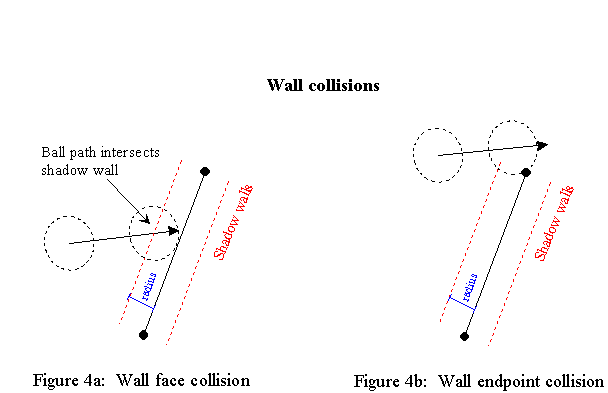That gives you a possible collision. Next, you need to decide if the ball actually hit the wall face, or if it passed beyond the end of the wall and possibly hit the endpoint.

To find if the ball hit a wall face, find the distance from the center of the ball to each endpoint of the shadow wall. If either distance is greater that the distance from one endpoint to the other then the center missed the wall face.

To find if the ball hit the endpoint of a wall, treat the wall endpoint as an infinitely massive ball of zero size and go through the ball collision above (see figure 4b).

## Simultaneous collisions

Again, we haven't implemented this yet. There are two likely candidates for this.

First, go through each pair of objects and find the pair lowest time of collision. Perform that collision and then find the next lowest time, taking into account the new velocities of the objects.

Or, sort the times and step through them in that order. Resort all objects that are close enough to be affected by each collision as they happen.

Wrapping it up

I hope you found this description useful. I realize it's ended up a bit on the terse side. Hopefully the PCX files will help you visualize what I'm trying to describe. If anyone has questions, feedback, suggestions or critique please feel free to contact me, Drake Christensen mighty@mightydrake.com.

This file owes its existence to David Bollinger. He and I spent over a month brainstorming this surprizingly obscure problem in the GAMDEV forum on Compuserve. His CBENCH example is available in GAMDEV. Obviously, any mistakes or obfuscation in this text are entirely my fault.

Another who provided needed assistance is Csaba Markus. We found his CAROM simulator at just the right time to convince ourselves that we were heading down (one of) the right path.

I can't give a specific bibliography, but if you want to research this in texts, my suggestion is to visit a local college library and look for mechanics and physics texts circa 1910 or so. I was unable to find anything more recent than those. Apparently, the academic community doesn't consider this an interesting subject anymore. :-)

Related files:
CBENCH.ZIP - Collision test bench by David Bollinger
BUMP.ZIP - Ricochet example by Gordon D. Griesel
CAROM.ZIP - Carom simulator by Csaba Markus
CLDTHD.ZIP - Thread on collision physics by (mostly) David Bollinger and Drake Christensen

##### Please email comments, typos, errors, dead links, and any suggestions to webmaster@mightydrake.com. (Privacy statement) Copyright © 1997-2007 Mighty Drake, Inc. All rights reserved. Last modified: January 06, 2004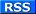Best viewed with: Hosted by: Composed with: In association  with: Fight Spam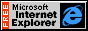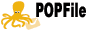Opera or Mozilla or Explorer or Netscape Site 5 FrontPage Amazon.com Spamcop.net &  Popfile & Greylisting